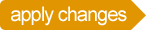## Expressions

There are three types of expressions:

1. Expressions that manipulate or test the values of sprites, variables or data sets
2. Math. Simple math equations can be used as values for your parameters. They support */+-% and paranthesis. If you are familiar with Javascript, you can also use the Math class methods: "where x=Math.min(30,s.x)"
3. References. These refer to the values held by other sprites. This lets you use the alpha value from ball, and apply it to fan: "fan.alpha=ball.alpha"

## Random

editable## Math

editable## References

editable